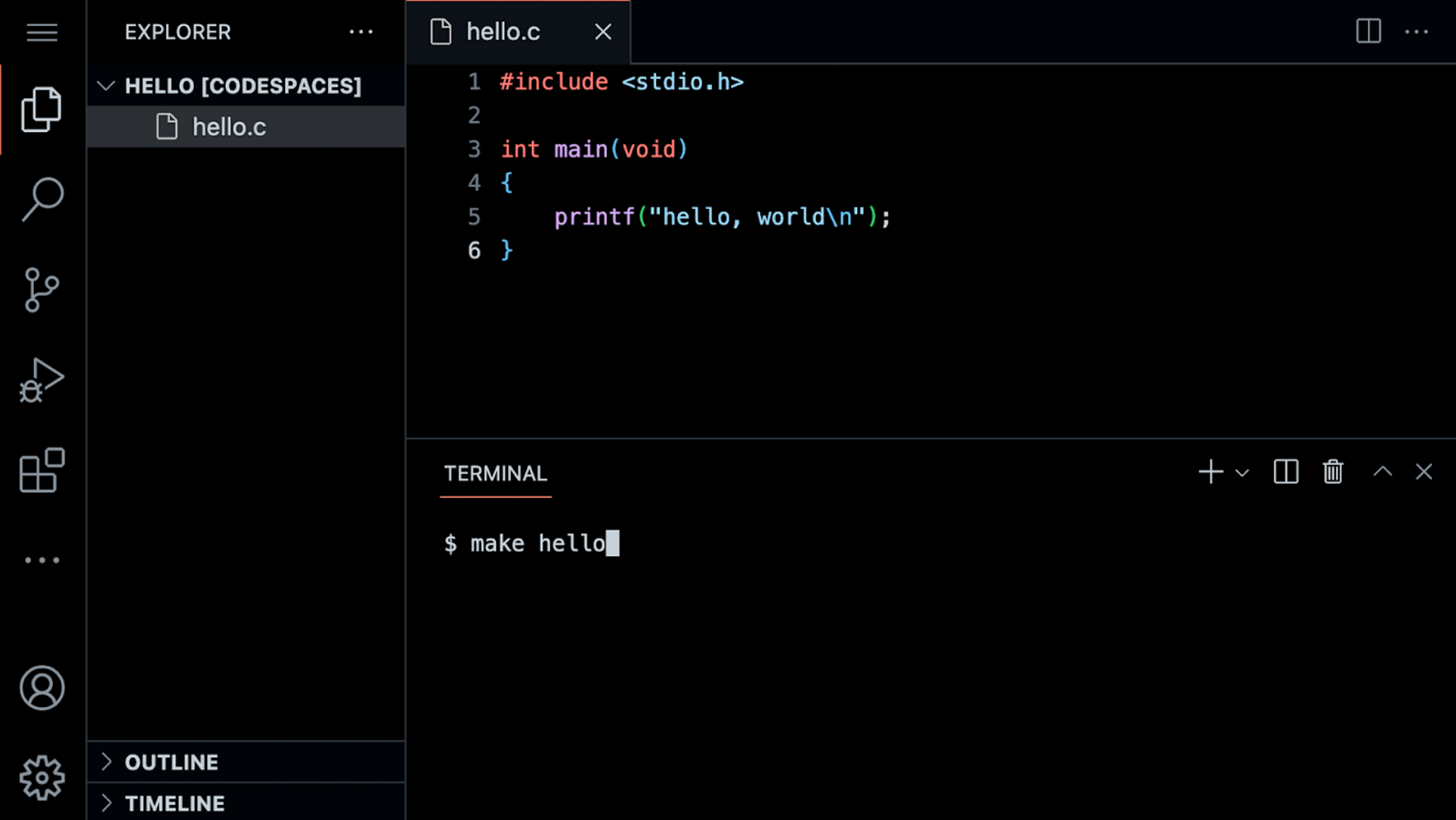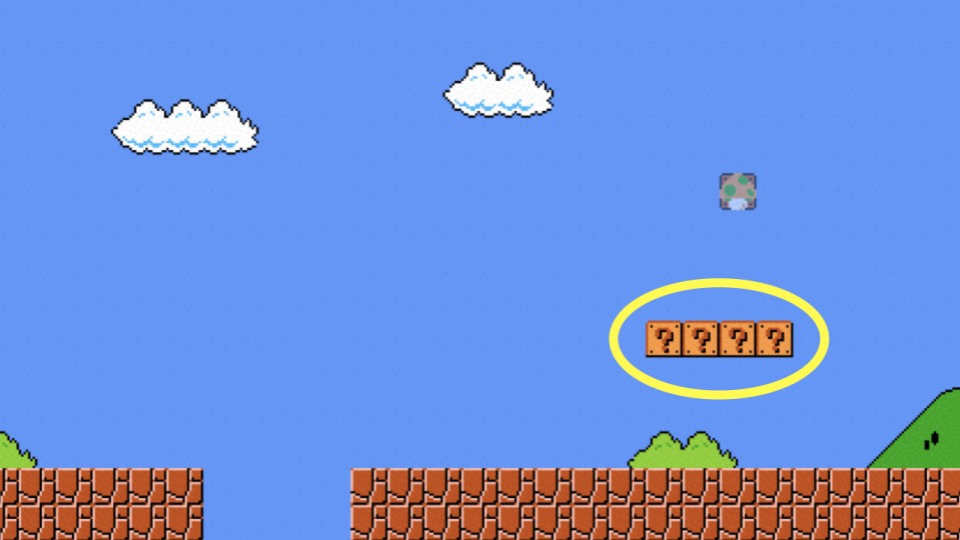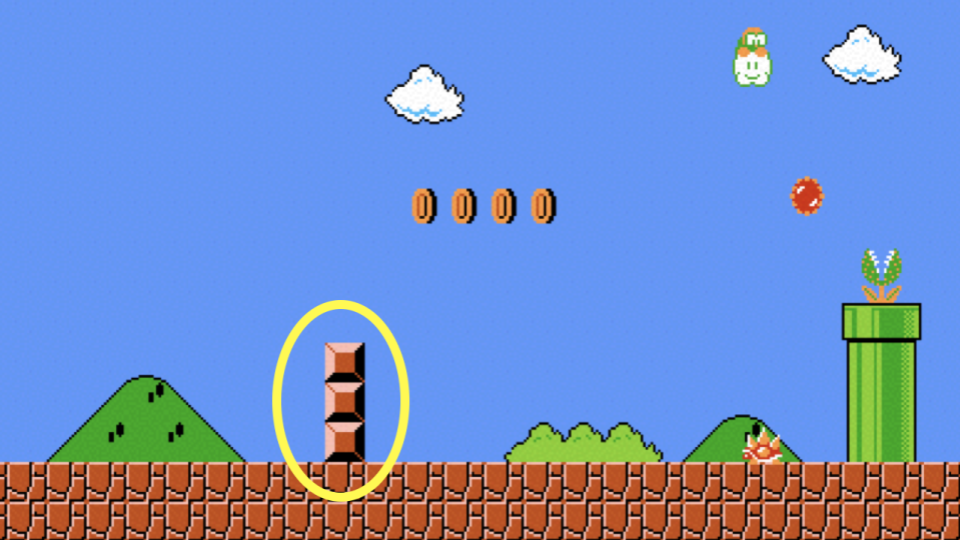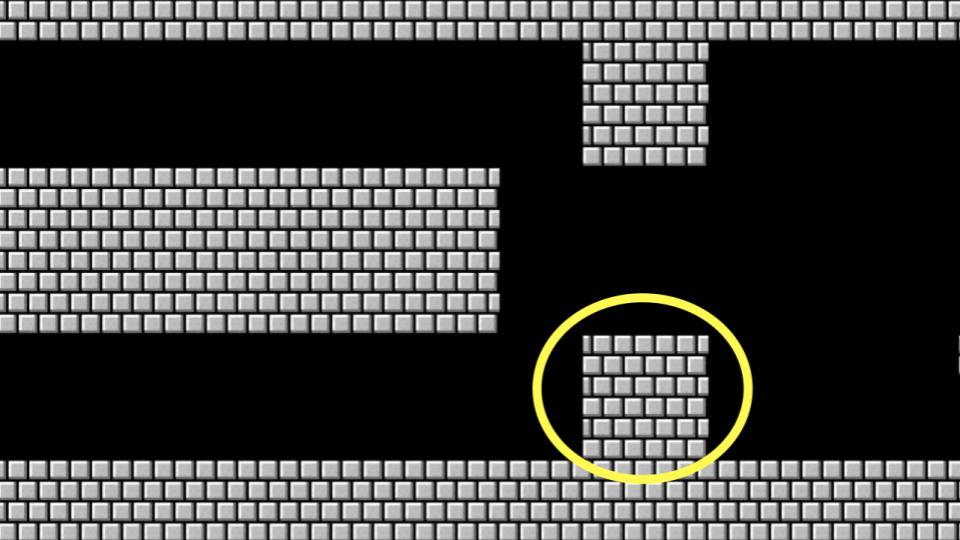# Lecture 1

## Welcome!

• In our previous session, we learned about Scratch, a visual programming language.
• Indeed, all the essential programming concepts presented in Scratch will be utilized as you learn how to program any programming language.
• Recall that machines only understand binary. Where humans write source code, a list of instructions for the computer that is human readable, machines only understand what we can now call machine code. This machine code is a pattern of ones and zeros that produces a desired effect.
• It turns out that we can convert source code into machine code using a very special piece of software called a compiler. Today, we will be introducing you to a compiler that will allow you to convert source code in the programming language C into machine code.
• Today, in addition to learning about how to code, you will be learning about how to write good code.
• Code can be evaluated upon three axes. First, correctness refers to “does the code run as intended?” Second, design refers to “how well is the code designed?” Finally, style refers to “how aesthetically pleasing and consistent is the code?”

## Hello World

• The compiler that is utilized for this course is Visual Studio Code, affectionately referred to as , which can be accessed via that same url, or simply as *VS Code.*
• One of the most important reasons we utilize VS Code is that it has all the software required for the course already pre-loaded on it. This course and the instructions herein were designed with VS Code in mind. Best always to utilize VS Code for assignments in this course.
• You can open VS Code at code.cs50.io.
• The compiler can be divided into a number of regions:Notice that there is a file explorer on the left side where you can find your files. Further, notice that there is a region in the middle called a text editor where you can edit your program. Finally, there is a command line interface, known as a CLI, command line, or terminal window where we can send commands to the computer in the cloud.

• We can build your first program in C by typing code hello.c into the terminal window. Notice that we deliberately lowercased the entire filename and included the .c extension. Then, in the text editor that appears, write code as follows:

#include <stdio.h>

int main(void)
{
printf("hello, world\n");
}


Note that every single character above serves a purpose. If you type it incorrectly, the program will not run.

• Clicking back in the terminal window, you can compile your code by executing make hello. Notice that we are omitting .c. make is a compiler that will look for our hello.c file and turn it into a program called hello. If executing this command results in no errors, you can proceed. If not, double-check your code to ensure it matches the above.
• Now, type ./hello and your program will execute saying hello, world.
• Now, open the file explorer on the left. You will notice that there is now both a file called hello.c and another file called hello. hello.c is able to be read by the compiler: It’s where your code is stored. hello is an executable file that you can run, but cannot be read by the compiler.
• Let’s look at our code more carefully:

#include <stdio.h>

int main(void)
{
printf("hello, world\n");
}


Notice that our code is highlighted using syntax highlighting.

## Functions

• In Scratch, we utilized the say block to display any text on the screen. Indeed, in C, we have a function called printf that does exactly this.
• Notice our code already invokes this function:

printf("hello, world\n");


Notice that the printf function is called. The argument passed to printf is ‘hello, world\n’. The statement of code is closed with a ;.

• A common error in C programming is the omission of a semicolon. Modify your code as follows:

#include <stdio.h>

int main(void)
{
printf("hello, world\n")
}


Notice the semicolon is now gone.

• In your terminal window, run make hello. You will now be met with numerous errors! Placing the semicolon back in the correct position and running make hello again, the errors go away.
• Notice also the special symbol \n in your code. Try removing those characters and making your program again by executing make hello. Typing ./hello in the terminal window, how did your program change?
• Restore your program to the following:

#include <stdio.h>

int main(void)
{
printf("hello, world\n");
}


Notice the semicolon and \n have been restored.

• The statement at the start of the code #include <stdio.h> is a very special command that tells the compile that you want to use the capabilities of library called stdio.h. This allows you, among many other things, to utilize the printf function. You can read about all the capabilities of this library on the Manual Pages.
• It turns out that CS50 has its own library called cs50.h. Let’s use this library in your program.

## Variables

• Recall that in Scratch, we had the ability to ask the user “What’s your name?” and say “hello” with that name appended to it.
• In C, we can do the same. Modify your code as follows:

#include <cs50.h>
#include <stdio.h>

int main(void)
{
}


Notice that #include <cs50.h> has been added to the top of your code. The get_string function is used to get a string from the user. Then, the variable answer is passed to the printf function. %s tells the printf function to prepare itself to receive a string.

• answer is a special holding place we call a variable. answer is of type string and can hold any string within it. There are many data types, such as int, bool, char, and many others.
• Running make hello again in the terminal window, you can run your program by typing ./hello. The program now asks for your name and then says hello with your name attached.

## Conditionals

• Another building block you utilized within Scratch was that of conditionals. For example, you might want to do one thing if x is greater than y. Further, you might want to do something else if that condition is not met.
• In the terminal window, type code compare.c and write code as follows:

#include <cs50.h>
#include <stdio.h>

int main(void)
{
int x = get_int("What's x? ")
int y = get_int("What's y? ")

if (x < y)
{
printf("x is less than y\n")
}
}


Notice that we create two variables, an int or integer called x and another called y. The values of these are populated using the get_int function.

• You can run your code by executing make compare in the terminal window, followed by ./compare. If you get any error messages, check your code for errors.
• We can improve your program by coding as follows:

#include <cs50.h>
#include <stdio.h>

int main(void)
{
int x = get_int("What's x? ")
int y = get_int("What's y? ")

if (x < y)
{
printf("x is less than y\n");
}
else if (x > y)
{
printf("x is greater than y\n");
}
else
{
printf("x is equal to y\n");
}
}


Notice that all potential outcomes are now accounted for.

• You can re-make and re-run your program and test it out.
• Considering another data type called a char we can start a new program by typing code agree.c into the terminal window. In the text editor, write code as follows:

#include <cs50.h>
#include <stdio.h>

int main(void)
{
// Prompt user to agree
char c = get_char("Do you agree? ");

// Check whether agreed
if (c == 'Y' || c == 'y')
{
printf("Agreed.\n");
}
else if (c == 'N' || c == 'n')
{
printf("Not agreed.\n");
}
}


Notice that single quotes are utilized for single characters. Further, notice that == ensure that something is equal to something else, where a single equal sign would have a very different function in C. Finally, notice that || effectively means or.

• You can test your code by typing make agree into the terminal window, followed by ./agree.

## Loops

• We can also utilize the loops building block from Scratch in our C programs.
• In your terminal window, type code meow.c and write code as follows:

#include <stdio.h>

int main(void)
{
printf("meow\n");
printf("meow\n");
printf("meow\n");
}


Notice this does as intended but has an opportunity for better design.

• We can improve our program by modifying your code as follows:

#include <stdio.h>

int main(void)
{
int i = 0;
while (i < 3)
{
printf("meow\n");
i++;
}
}


Notice that we create an int called i and assign it the value 0. Then, we create a while loop that will run as long as i < 3. Then, the loop runs. Every time 1 is added to i using the i++ statement.

• Similarly, we can implement a count-down of sorts by modifying our code as follows:

#include <stdio.h>

int main(void)
{
int i = 3;
while (i > 0)
{
printf("meow\n");
i--;
}
}


Notice how our counter i is started at 3. Each time the loop runs, it will reduce the counter by 1. Once the counter is less than zero, it will stop the loop.

• We can further improve the design using a for loop. Modify your code as follows:

#include <stdio.h>

int main(void)
{
for (int i = 0; i < 3; i++)
{
printf("meow\n");
}
}


Notice that the for loop includes three arguments. The first argument int i = 0 starts our counter at zero. The second argument i < 3 is the condition that is being checked. Finally, the argument i++ tells the loop to increment by one each time the loop runs.

• We can even loop forever using the following code:

#include <cs50.h>
#include <stdio.h>

int main(void)
{
while (true)
{
printf("meow\n");
}
}


Notice that true will always be the case. Therefore, the code will always run. You will lose control of your terminal window by running this code. You can break from an infinite by hitting control-C on your keyboard.

## Linux and the Command Line

• Linux is an operating system that is accessible via the command line in the terminal window in VS Code.
• Some common command-line arguments we may use include:
• cd, for changing our current directory (folder)
• cp, for copying files and directories
• ls, for listing files in a directory
• mkdir, for making a directory
• mv, for moving (renaming) files and directories
• rm, for removing (deleting) files
• rmdir, for removing (deleting) directories
• The most commonly used is ls which will list all the files in the current directory or directory. Go ahead and type ls into the terminal window and hit enter. You’ll see all the files in the current folder.
• Another useful command is mv, where you can move a file from one file to another. For example, you could use this command to rename Hello.c (notice the uppercase H) to hello.c by typing mv Hello.c hello.c.
• You can also create folders. You can type mkdir pset1 to create a directory called pset1.
• You can then use cd pset1 to change your current directory to pset1.

## Mario

• Everything we’ve discussed today has focused on various building-blocks of your work as a programmer.
• The following will help you orient toward working on a problem set for this class in general: How does one approach a computer science related problem?
• Imagine we wanted to emulate the visual of the game Super Mario Bros. Considering the four question-blocks pictured, how could we create code that roughly represents these four horizontal blocks?• In the terminal window, type code mario.c and code as follows:

#include <stdio.h>

int main(void)
{
for (int i = 0; i < 4; i++)
{
printf("?");
}
printf("\n");
}


Notice how four question marks are printed here using a loop.

• Similarly, we can apply this same logic to be able to create three vertical blocks.• To accomplish this, modify your code as follows:

#include <stdio.h>

int main(void)
{
for (int i = 0; i < 3; i++)
{
printf("#\n");
}
}


Notice how three vertical bricks are printed using a loop.

• What if we wanted to combine these ideas to create a three-by-three group of blocks?• We can follow the logic above, combining the same ideas. Modify your code as follows:

#include <stdio.h>

int main(void)
{
for (int i = 0; i < 3; i++)
{
for (int j = 0; j < 3; j++)
{
printf("#");
}
printf("\n");
}
}


Notice that one loop is inside another. The first loop defines what vertical row is being printed. For each row, three columns are printed. After each row, a new line is printed.

• What if we wanted to ensure that the number of blocks to be constant, that is, unchangeable? Modify your code as follows:

int main(void)
{
const int n = 3;
for (int i = 0; i < n; i++)
{
for (int j = 0; j < n; j++)
{
printf("#");
}
printf("\n");
}


Notice how n is now a constant. It can never be changed.

• As illustrated earlier in this lecture, we can make our code prompt the user for the size of the grid. Modify your code as follows:

#include <cs50.h>
#include <stdio.h>

int main(void)
{
int n = get_int("Size: ");

for (int i = 0; i < n; i++)
{
for (int j = 0; j < n; j++)
{
printf("#");
}
printf("\n");
}
}


Notice that get_int is used to prompt the user.

• A general piece of advice within programming is that you should never fully trust your user. They will likely misbehave, typing incorrect values where they should not. We can protect our program from bad behavior by checking to make sure the user’s input satisfies our needs. Modify your code as follows:

#include <cs50.h>
#include <stdio.h>

int main(void)
{
int n;
do
{
n = get_int("Size: ");
}
while (n < 1);

for (int i = 0; i < n; i++)
{
for (int j = 0; j < n; j++)
{
printf("#");
}
printf("\n");
}
}


Notice how the user is continuously prompted for the size until the user’s input is 1 or greater.

• Comments are fundamental parts of a computer program, where you leave explanatory remarks to yourself and others that may be collaborating with you regarding your code.
• All code you create for this course must include robust comments.
• Typically each comment is a few words or more, providing the reader an opportunity to understand what is happening in a specific block of code. Further, such comments serve as a reminder for you later when you need to revise your code.
• Comments involve placing // into your code, followed by a comment. Modify your code as follows to integrate comments:

#include <cs50.h>
#include <stdio.h>

int main(void)
{
// Get size of grid
int n;
do
{
n = get_int("Size: ");
}
while (n < 1);

// Print grid of bricks
for (int i = 0; i < n; i++)
{
for (int j = 0; j < n; j++)
{
printf("#");
}
printf("\n");
}
}


Notice how each comment begins with a //.

## Abstraction

• Abstraction is the art of simplifying our code such that it deals with smaller and smaller problems.
• Looking at your code, you can see how two essential problems in our code are get size of grid and print grid of bricks.
• We can abstract away these two problems into separate functions. Modify your code as follows:

#include <cs50.h>
#include <stdio.h>

int get_size(void);
void print_grid(int n);

int main(void)
{
int n = get_size();
print_grid(n);
}

int get_size(void)
{
int n;
do
{
n = get_int("Size: ");
}
while (n < 1);
return n;
}

void print_grid(int n)
{
for (int i = 0; i < n; i++)
{
for (int j = 0; j < n; j++)
{
printf("#");
}
printf("\n");
}
}


Notice that we have three functions now. First, we have the main function that calls two other functions called get_size and print_grid. Second, we have a second function called get_size which includes the exact code we had to accomplish this task prior. Third, we have another function called print_grid that prints the grid. Because we abstracted away the essential problems within our program, our main function is very short.

## Operators and Types

• Operators refer to the mathematical operations that are supported by your compiler. In C, these mathematical operators include:

• + for addition
• - for subtraction
• * for multiplication
• / for division
• % for remainder
• Types refer to the possible data that can be stored within a variable. For example, a char is designed to accommodate a single character like a or 2.
• Types are very important because each type has specific limits. For example, because of the limits in memory, the highest value of an int can be 4294967296.
• Types with which you might interact during this course include:

• bool, a Boolean expression of either true or false
• char, a single character like a or 2
• double, a floating-point value with more digits than a float
• float, a floating-point value, or real number with a decimal value
• int, integers up to a certain size, or number of bits
• long, integers with more bits, so they can count higher than an int
• string, a string of characters
• You can implement a calculator in C. In your terminal, type code calculator.c and write code as follows:

#include <cs50.h>
#include <stdio.h>

int main(void)
{
// Prompt user for x
int x = get_int("x: ");

// Prompt user for y
int y = get_int("y: ");

printf("%i\n", x + y);
}


Notice how the get_int function is utilized to obtain an integer from the user twice. One integer is stored in the int variable called x. Another is stored in the int variable called y. Then, the printf function prints the value of x + y, designated by the %i symbol.

• As you are coding, pay special attention to the types of variables you are using to avoid problems within your code.

## Summing Up

In this lesson, you learned how to apply the building blocks you learned in Scratch to the C programming language. You learned…

• How to create your first program in C.
• Predefined functions that come natively with C and how to implement your own functions.
• How to use variables, conditionals, and loops.
• How to use the Linux command line.
• How to approach problem-solving for a computer science problem.